# Calculate Integer Part of a Number – Excel & Google Sheets

This tutorial will demonstrate how to calculate the integer portion of a number in Excel and Google Sheets.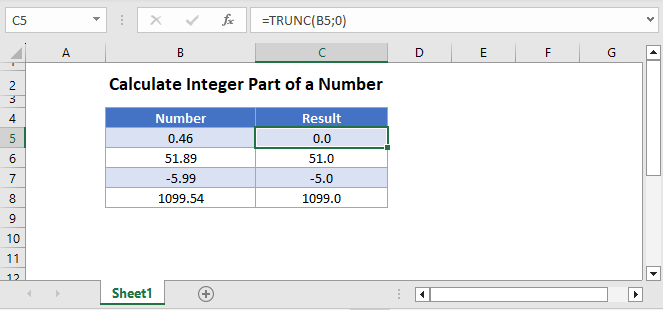## Integer Portion of Number

To calculate the integer portion of a number, use the TRUNC Function to “truncate” off the decimal.

``=TRUNC(B3,0)``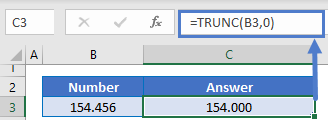The TRUNC Function simply cuts off the decimal, it does not round.

### INT and ROUND Functions

You might be wondering about the INT or ROUND Functions.

The INT Function will work exactly the same as TRUNC for positive numbers, but for negative numbers INT rounds numbers down away from zero.

``=INT(B3)``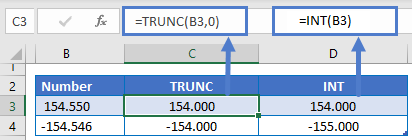The ROUND Function rounds number, which can give different answers than the other functions.

``=ROUND(B3,0)``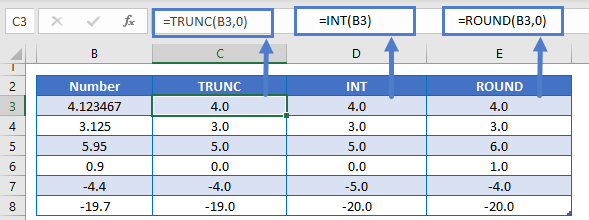## Calculate Integer Part of a Number in Google Sheets

All of the above examples work exactly the same in Google Sheets as in Excel.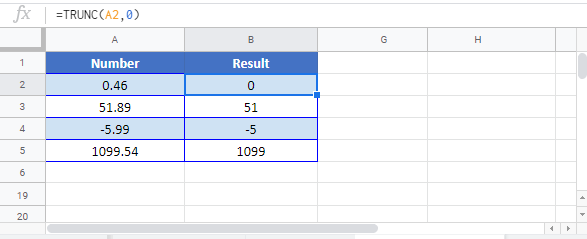### Excel Practice Worksheet

Practice Excel functions and formulas with our 100% free practice worksheets!